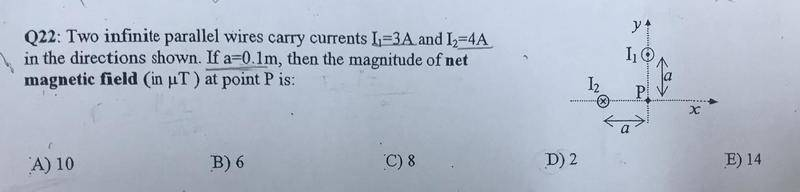# Find the magnetic field at a point

## Homework StatementB=(μ0*I)/(2πr)

## The Attempt at a Solution

B2 = (4*4*10-7)/(2*0.1)
= 8 μT
B1 = (3*4*10-7)/(2*0.1)
= 6μT
Could I know how to determine the directions ?

#### Attachments

BvU
Homework Helper
Know about the right-hand rule ?

The directions matter (to some extent), but
do the signs matter ? They are only asking for a magnitude ...

Know about the right-hand rule ?
Yeah

BvU
Homework Helper
Yeah
So draw the arrows to find how you have to add them.

So draw the arrows to find how you have to add them.
B2 = 8μT (-j) , because the direction of I2 is (-i).
B1 = 6μT (i) , because the direction of I1 is (j)
So , Bnet = √(82+62) = 10 μT ?

BvU
Homework Helper
the direction of I2 is (-i)
I'd vote for ##-\hat k##I'd vote for ##-\hat k##So , B2 (##-\hat j##)
B1 (##\hat i##)

BvU
I agree. (I also agreed with #5, except for the directions of the currents)
•